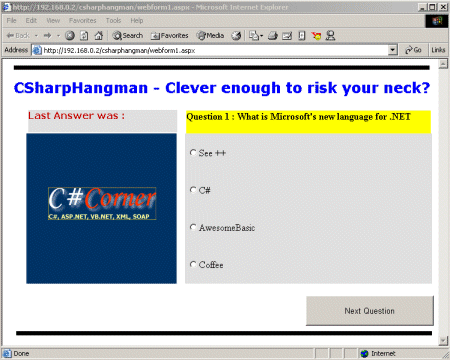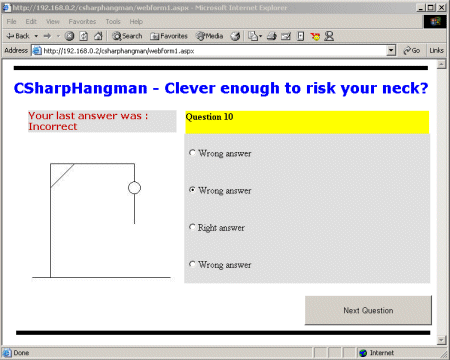Post

# Hangman: Using GDI+ in ASP.NET Applications

• 31.9k
• 0
• 0

This example shows how you can combine GDI+ commands on a web form.

After experimenting with GDI+ in previous articles I turned my attention to ASP.NET and thought what if you could use GDI+ commands on a webform.

Of course the code behind a webform runs on the server so unfortunately I cant draw on the form and expect it to show on the Internet Browser. But as always with .NET there is a way.

My example code implements a web page which is the game of hangman written in C#. For those that are not familiar the idea is to answer questions and every time you get one wrong you get closer to being hanged.

In my version you will see that I draw on an Image control on the web form and then save that image back to the hard drive. On doing this the server automatically updates the client with the new image.

Here is the first screen :As you can see I am using an Image control and asking a question. In the source code you will see I am storing the questions and answers in an array and also storing an indicator for the correct answer.

When you click your answer and then hit Next Question I compare your answer and check it against the correct one. If you chose correctly we simply move to the next question. If you chose wrongly we start drawing the hangman! Here is a screenshot after I have answered incorrectly a few times.Heres how its done.

Firstly I have to create an image for the Image control on the web page like this :

Bitmap b=new Bitmap(250,250);

b.Save("c:\\inetpub\\wwwroot\\csharphangman\\temp5.jpeg",ImageFormat.Jpeg);

System.Drawing.Image i=System.Drawing.Image.FromFile("c:\\inetpub\\wwwroot\\csharphangman\\temp5.jpeg");

Graphics g=Graphics.FromImage(i);
g.Clear(Color.White);

You can see I create an Image class of the Bitmap and then a Graphics class of the Image class. Once I have the Graphics class I can start drawing lines.

Depending on how many questions you get wrong I draw a number of lines using custom methods like this.

private Graphics DisplayLevel9(Graphics g)
{
Pen p =
new Pen(Color.Black);
g.DrawLine(p,10,240,240,240);
g.DrawLine(p,40,240,40,50);
g.DrawLine(p,40,50,180,50);
g.DrawLine(p,80,50,40,90);
g.DrawLine(p,180,50,180,80);
g.DrawEllipse(p,170,80,20,20);
g.DrawLine(p,180,100,180,150);
g.DrawLine(p,180,150,170,170);
g.DrawLine(p,180,150,190,170);
g.DrawLine(p,180,110,170,120);
g.DrawLine(p,180,110,190,120);
return(g);
}

The code above draws the entire hangman. You can see I have passed in the Graphics class for my Image, I then draw and then return the Graphics class.

One done I can then save the Image to a new file and point the Image control on the webform to the new Image. When this is done the form is reloaded with the new image. The result is I am using GDI+ in a webform!

This code shows me resetting the image for the Image control on the webform

i.Save("c:\\inetpub\\wwwroot\\csharphangman\\john2.jpeg",ImageFormat.Jpeg);

b.Dispose();
i.Dispose();
g.Dispose();

this.Image1.ImageUrl="http://192.168.0.2/csharphangman/john2.jpeg";

Sessions

In order to track which questions I have got write or wrong I use sessions. These are created and updated as simply as doing this :

Session["Question"]="1";
Session["Wrong"]="0";

Sessions are a valuable tool for storing program state. In my case my code checks which question we are at and re-populates the form accordingly. Note I can change the form controls and ASP.NET takes case of sending them back to the Browser.

End of the program.

Once you reach the end of the program either by winning or losing I hide the Next Question button and then make visible a hyperlink back to www.csharp-corner.com

Conclusions

With .NET it is easy to create variables and classes on the fly rather than the old days where you declared everything at the start of the code. When I had completed the program I found I was doing things like declaring new Pens on each line of code to draw a line rather than just declare one Pen at the start of the code. I have read that you should create variables as you use them but for me this means you are not planning your code. I actually noticed a speed up when I moved to only declaring one Pen.

Lastly, I have stored all my questions in an array in the code itself. Of course these questions could have easily come from a SQL database where I could randomly choose say 15 questions from a database of hundreds.

//CSharpHangman
//Creates a hangman quiz game as a web form.
//Written 09/10/01 By John O'Donnell - wincepro@hotmail.com
using System;
using System.Collections;
using System.ComponentModel;
using System.Data;
using System.IO;
using System.Drawing;
using System.Drawing.Imaging;
using System.Web;
using System.Web.SessionState;
using System.Web.UI;
using System.Web.UI.WebControls;
using System.Web.UI.HtmlControls;
namespace CSharpHangman
{
/// <summary>
/// Summary description for WebForm1.
/// </summary>
public class WebForm1 : System.Web.UI.Page
{
string [][] questionarray= new string [];
protected System.Web.UI.WebControls.Image Image1;
protected System.Web.UI.WebControls.Label Label2;
protected System.Web.UI.HtmlControls.HtmlInputButton Button1;
protected System.Web.UI.WebControls.Label Label3;
protected System.Web.UI.WebControls.Label Label1;
public WebForm1()
{
Page.Init +=
new System.EventHandler(Page_Init);
}
private void Page_Load(object sender, System.EventArgs e)
{
// Put user code to initialize the page here
initquestions();
Label2.Text=questionarray;
}
private void Page_Init(object sender, EventArgs e)
{
//
// CODEGEN: This call is required by the ASP.NET Web Form Designer.
//
InitializeComponent();
}
#region Web Form Designer generated code
/// <summary>
/// Required method for Designer support - do not modify
/// the contents of this method with the code editor.
/// </summary>
private void InitializeComponent()
{
this.Button1.ServerClick += new System.EventHandler(this.Button1_ServerClick);
}
#endregion
private void RadioButton1_CheckedChanged(object sender, System.EventArgs e)
{
}
private void RadioButtonList1_SelectedIndexChanged(object sender, System.EventArgs e)
{
}
private void Button1_ServerClick(object sender, System.EventArgs e)
{
if(Session.Count==0)
{
Session["Question"]="1";
Session["Wrong"]="0";
string level=Session["Question"].ToString();
Label2.Text=questionarray;
}
int question = Convert.ToInt32(Session["Question"].ToString());
{
int s=Convert.ToInt32(Session["Question"].ToString());
s++;
UpdateFormQuestions(s);
}
else
{
int i=Convert.ToInt32(Session["Wrong"].ToString());
i++;
Session["Wrong"]=Convert.ToString(i);
int s=Convert.ToInt32(Session["Question"].ToString());
s++;
UpdateFormQuestions(s);
}
string a;
//work out which hangman graphic to display
a=Session["Wrong"].ToString();
a=UpdateGraphic(a);
//check to see if user has finished the quiz or got to many wrong answers
if ((Convert.ToInt32(a))==12)
{
this.Image1.ImageUrl=http://192.168.0.2/csharphangman/winner.gif;
Button1.Visible=
false;
true;
}
if ((Convert.ToInt32(Session["Wrong"])==9))
{
this.Image1.ImageUrl=http://192.168.0.2/csharphangman/hangman.gif;
Button1.Visible=
false;
true;
}
}
public void UpdateFormQuestions(int qnumber)
{
Session["Question"]=Convert.ToString(qnumber);
Label2.Text=questionarray[qnumber];
}
public void initquestions()
{
questionarray=
new string[] {"Question 1 : What is Microsoft's new language for .NET","See ++","C#","AwesomeBasic","Coffee","3"};
questionarray=
new string[] {"Question 2 : Which of these is not a method of the Graphics class","DrawArc","DrawTriangle","DrawIcon","DrawEllipse","3"};
questionarray=
new string[] {"Question 3 : Which person is an architect of .NET and C#","Larry Ellison","Bill Gates","Kermit the Frog","Anders Hejlsberg","5"};
questionarray=
new string[] {"Question 4 : Which is not a .NET Framework class?","Image","Photo","Bitmap","Graphics","3"};
questionarray=
new string[] {"Question 5 : Which programming language has been around the longest?","Java","Cobol","C++","C#","5"};
questionarray=
questionarray=
questionarray=
questionarray=
questionarray=
questionarray=
questionarray=
}
public string UpdateGraphic(string sess)
{
Bitmap b=
new Bitmap(250,250);
b.Save("c:\\inetpub\\wwwroot\\csharphangman\\temp5.jpeg",ImageFormat.Jpeg);
System.Drawing.Image i=System.Drawing.Image.FromFile("c:\\inetpub\\wwwroot\\csharphangman\\temp5.jpeg");
Graphics g=Graphics.FromImage(i);
g.Clear(Color.White);
//display appropriate hangman graphic
switch (sess)
{
case "0" :
g=DisplayLevel0(g);
break;
case "1" :
g=DisplayLevel1(g);
break;
case "2" :
g=DisplayLevel2(g);
break;
case "3" :
g=DisplayLevel3(g);
break;
case "4" :
g=DisplayLevel4(g);
break;
case "5" :
g=DisplayLevel5(g);
break;
case "6" :
g=DisplayLevel6(g);
break;
case "7" :
g=DisplayLevel7(g);
break;
case "8" :
g=DisplayLevel8(g);
break;
case "9" :
g=DisplayLevel9(g);
break;
}
i.Save("c:\\inetpub\\wwwroot\\csharphangman\\john2.jpeg",ImageFormat.Jpeg);
b.Dispose();
i.Dispose();
g.Dispose();
this.Image1.ImageUrl=http://192.168.0.2/csharphangman/john2.jpeg;
File.Delete("c:\\inetpub\\wwwroot\\csharphangman\\temp5.jpeg");
string a=Session["Question"].ToString();
return(a);
}
private Graphics DisplayLevel0(Graphics g)
{
this.Image1.ImageUrl=http://192.168.0.2/csharphangman/logo.jpeg;
return(g);
}
private Graphics DisplayLevel1(Graphics g)
{
Pen p =
new Pen(Color.Black);
g.DrawLine(p,10,240,240,240);
return(g);
}
private Graphics DisplayLevel2(Graphics g)
{
Pen p =
new Pen(Color.Black);
g.DrawLine(p,10,240,240,240);
g.DrawLine(p,40,240,40,50);
return(g);
}
private Graphics DisplayLevel3(Graphics g)
{
Pen p =
new Pen(Color.Black);
g.DrawLine(p,10,240,240,240);
g.DrawLine(p,40,240,40,50);
g.DrawLine(p,40,50,180,50);
return(g);
}
private Graphics DisplayLevel4(Graphics g)
{
Pen p =
new Pen(Color.Black);
g.DrawLine(p,10,240,240,240);
g.DrawLine(p,40,240,40,50);
g.DrawLine(p,40,50,180,50);
g.DrawLine(p,80,50,40,90);
return(g);
}
private Graphics DisplayLevel5(Graphics g)
{
Pen p =
new Pen(Color.Black);
g.DrawLine(p,10,240,240,240);
g.DrawLine(p,40,240,40,50);
g.DrawLine(p,40,50,180,50);
g.DrawLine(p,80,50,40,90);
g.DrawLine(p,180,50,180,80);
return(g);
}
private Graphics DisplayLevel6(Graphics g)
{
Pen p =
new Pen(Color.Black);
g.DrawLine(p,10,240,240,240);
g.DrawLine(p,40,240,40,50);
g.DrawLine(p,40,50,180,50);
g.DrawLine(p,80,50,40,90);
g.DrawLine(p,180,50,180,80);
g.DrawEllipse(p,170,80,20,20);
return(g);
}
private Graphics DisplayLevel7(Graphics g)
{
Pen p =
new Pen(Color.Black);
g.DrawLine(p,10,240,240,240);
g.DrawLine(p,40,240,40,50);
g.DrawLine(p,40,50,180,50);
g.DrawLine(p,80,50,40,90);
g.DrawLine(p,180,50,180,80);
g.DrawEllipse(p,170,80,20,20);
g.DrawLine(p,180,100,180,150);
return(g);
}
private Graphics DisplayLevel8(Graphics g)
{
Pen p =
new Pen(Color.Black);
g.DrawLine(p,10,240,240,240);
g.DrawLine(p,40,240,40,50);
g.DrawLine(p,40,50,180,50);
g.DrawLine(p,80,50,40,90);
g.DrawLine(p,180,50,180,80);
g.DrawEllipse(p,170,80,20,20);
g.DrawLine(p,180,100,180,150);
g.DrawLine(p,180,150,170,170);
g.DrawLine(p,180,150,190,170);
return(g);
}
private Graphics DisplayLevel9(Graphics g)
{
Pen p =
new Pen(Color.Black);
g.DrawLine(p,10,240,240,240);
g.DrawLine(p,40,240,40,50);
g.DrawLine(p,40,50,180,50);
g.DrawLine(p,80,50,40,90);
g.DrawLine(p,180,50,180,80);
g.DrawEllipse(p,170,80,20,20);
g.DrawLine(p,180,100,180,150);
g.DrawLine(p,180,150,170,170);
g.DrawLine(p,180,150,190,170);
g.DrawLine(p,180,110,170,120);
g.DrawLine(p,180,110,190,120);
return(g);
}
}

Recommended Free Ebook
Similar Articles